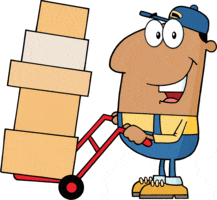# Math Worksheets Land

Math Worksheets For All Ages

# Math Worksheets Land

Math Worksheets For All Ages

Home > Grade Levels > High School Geometry >

# Rigid Motions to Transform Figures Worksheets

As we peel away more at this subject of geometry, we often find the need to communicate the movements of geometric figures either in free space or a coordinate plane. This can be for a variety of reasons and as we begin to use these skills in the real world, this language becomes critical. The Captains of huge naval vessel must be experts in this language to make sure that their ships are steered to safety during storms. When these movements do not change the actual shape of the figures, we call this type rigid motions. This selection of worksheets and lessons teaches you how to perform and raw these types of motions in the form of translations, rotations, reflections, and glide reflections.

### Aligned Standard: High School Geometry - HSG-CO.B.6

• Answer Keys - These are for all the unlocked materials above.

### Homework Sheets

It is all about thrown shapes on themselves.

• Homework 1 - The shape is a diamond and has 4(n) sides. Since n = 4 is even and l passes through the midpoints of two opposite sides, l is a line of symmetry. So, one answer is a reflection across l.
• Homework 2 - Rotating a parallelogram by 180° carries the parallelogram onto itself.
• Homework 3 - Since n = 5 is odd and l passes through the midpoints of two opposite sides, l is a line of symmetry. One answer is a reflection across l.

### Practice Worksheets

It's interesting to see what kids come up with when you ask them to describe geometric movements.

• Practice 1 - Describe the transformation that would carry this trapezoid onto itself.
• Practice 2 - Which type of transformation would carry this regular pentagon onto itself?
• Practice 3 - We focus a bit on triangles.

### Math Skill Quizzes

Most of the translations are one step, but there are a few two step translations here.

• Quiz 1 - Which of the transformations would carry this polygon onto itself?
• Quiz 2 - This is polygon based.
• Quiz 3 - More work on those pesky polygons.

### What are Rigid Motions to Transform Geometric Figures?The transformation of geometrical shapes in mathematics is an important concept. Any shape that changes its position or orientation from its actual position and orientation is a transformed geometric figure.

So, what are rigid motions in transformation? Rigid motions in geometrical transformation are the ones that do not affect the size, shape, or angles of a shape. As a result, this type of movement and change preserves the distances between the vertices of the original shape. It is important to not that transformation describe the transitioning portion of the change in the shape from a starting to ending location. This is helpful for identifying congruent figures because if a rigid motion is used to position a figure both the original and transformed figure are congruent.

There are three types of rigid motion in geometry, and these include translation, reflection, and rotation. The translation is the motion of a shape on the x- and the y-axis. The orientation of the translated figure stays the same, while its horizontal and vertical positions change. The reflection of a geometric shape is similar to how we see our reflections in the mirror. The dimensions, angles, and shape remain the same, but the object becomes inverted. The object is reflected over a reference or mirror line. The rotation is when a shape is turned about a center of rotation. Even in this case, the shape, size, and angles remain the same. There is also a form of rigid motion where an object moves from its beginning location to the same exact location and appears to not have moved at all. When this occurs it appears as if the object has not moved at all.

Unlock all the answers, worksheets, homework, tests and more!
Save Tons of Time! Make My Life Easier Now

## Thanks and Don't Forget To Tell Your Friends!

I would appreciate everyone letting me know if you find any errors. I'm getting a little older these days and my eyes are going. Please contact me, to let me know. I'll fix it ASAP.